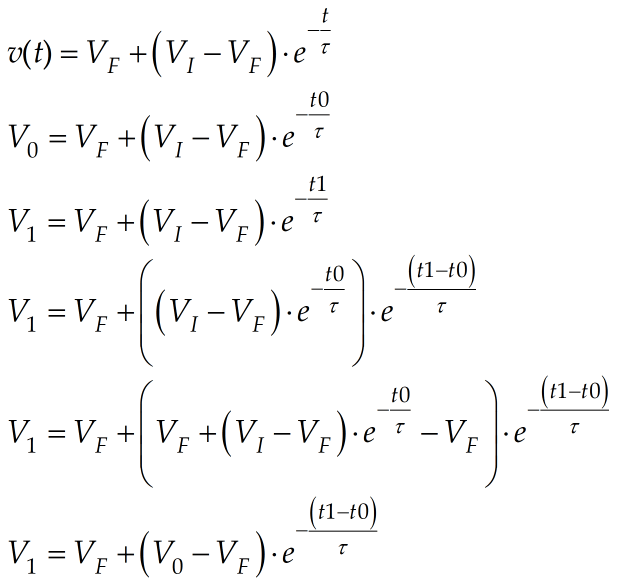# Estimating Exponential Time Constants

Quote of the Day

Engineering is achieving function while avoiding failure.

I have been presented with a large amount of experimental data from which I need to determine many exponential time constants. There are so many time constants to calculate that I need to automate the process.

I have data with dozens of exponential curves like shown in Figure 1.  I My plan is to estimate three points from every exponential curve: (t0, V0); (t1,V1); and (t2,V2). I will then determine the exponential time constant (τ) and the final voltage (VF) by fitting these three points to Equation 1.

 Eq. 1$\displaystyle v(t)={{V}_{F}}+\left( {{{V}_{I}}-{{V}_{F}}} \right)\cdot {{e}^{{-\frac{t}{\tau }}}}$

where

• VF is the final voltage of reached by the curve.
• VI is the initial voltage of the curve.
• τ is the exponential time constant that I need to compute.
• v(t) is the exponential voltage as a function of time.
• t is time.

Because I have two unknowns (τ, VF), I will need to solve two equations. In my case, I cannot see the point where the exponential curve begins – I will show below that it does not matter. In Figure 1, I select a reference point (t0, V0) and measure the two other points relative to V0, i.e., (V1, t1-t0) and (V2, t2-t0).

To find (τ, VF), I chose to use a nonlinear solver in Mathcad to solve the problem (Figure 2).

I should mention that it does not matter which point is chosen for the reference. You can prove this as shown below. In this derivation, I show that when you pick a reference V0, the point value of the point at t1 is driven by the time difference t1-t0.Figure 3: Derivation Showing that the Value of V1 is related only to the time difference from V0.

Thanks to this approach, I was able to determine all the exponential time constants quickly and accurately.

This entry was posted in General Mathematics. Bookmark the permalink.